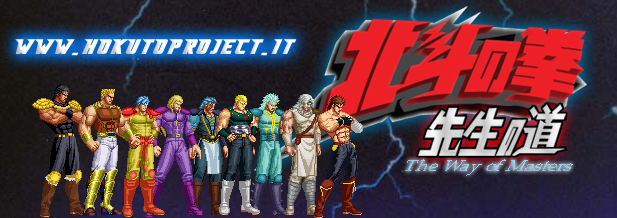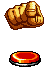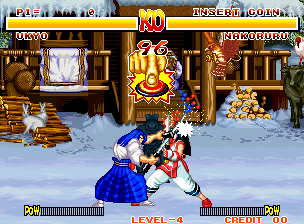The  pushpush mode  ( or Challenge mode )  it's a feature that you can see in a game called Samurai Spirit ( Samurai Shodown ) created by Snk, in this game when both player make the same attack and this attack collide... the 2 player go in the pushpush state...
The player who push for more time the attack buttons win.. and can hit the enemy ( enemy lost his weampon )
My code isn't exactly the same.. but only redone to work with mugen.

The first version of my code was used by the Hokuto Project .. but after many years.. I thing that's time for a n update.

To make the pushpush mode work we need a starter.. some thing that make all to start....

CNS for your character ( I suggest to you to download my evilsoul and to you as base of your work the player called "cavia" that you will find in the evilsouldark's rar - the file is esppc.cns ).
To avoid the annoying thing to go always in the pushpush mode I decide to use a var to delimit and limit this code.
The attack could be a simple punch or a kick... for this reason the pushpush code is activate only if our var(4) is egual or bigger ( as value ) of 9... in this way our gameplay isn't ruined...
Make attention to the priority... use the same value of this to avoid problems.
[State 280, VarRan]
type = VarRandom
trigger1 = time = 1
v = 4
range = 1,10

;In case our enemy haven't the pushpush code... or in case the var(4) is lower than 9, this "hidef" hit with a simple puch/kick the enemy.
[State 280, hd]
type = HitDef
triggerall = animelem = 2
trigger1 = enemy,var(0) = 0
trigger2 = enemy,var(0) = 59 && var(4) <= 8 && enemy, selfanimexist(28000)=1
attr = S, NA
animtype  = Medium
damage    = 57,5
guardflag = MA
pausetime = 12,12
sparkno = 1
sparkxy = -10,-83
hitsound   = 5,2
guardsound = 6,0
ground.type = High
ground.slidetime = 12
ground.hittime  = 13
ground.velocity = -5.5
air.velocity = -2.5,-4
priority = 4, hit

;This is the hitdef to active the pushpush, it works with attack collition or with a simple attack.
[State 280, hd]
type = HitDef
triggerall = enemy,var(0) = 59 && var(4) >= 9 && enemy, selfanimexist(28000)=1
trigger1 = AnimElem = 2
attr = S, NA
animtype  = Medium
damage    = 0
guardflag = MA
pausetime = 12,12
sparkno = 1
sparkxy = -10,-83
hitsound   = 5,2
guardsound = 6,0
ground.type = High
ground.slidetime = 12
ground.hittime  = 13
ground.velocity = -5.5
air.velocity = -2.5,-4
priority = 7, hit
p1stateno = 28000
p2stateno = 28001
p1facing = 1
p2facing = 1
kill=0

28000 <- is the tru pushpush statedef
28001 <- is only a transitional state from.. custmonstate to selfstate for the enemy

;Pushpush the tru code
[Statedef 28000]
type    = S
movetype= A
physics = S
juggle  = 4
ctrl = 0
velset = 0,0
anim = 28000  ;<-- the basic and essential animation no collitionbox in this anim
sprpriority = -1

;This 2 vars are setting to define who is the winner.. I choose to set 2 var for the 2 direction ( player facing is to right or to left ).
[state 28000, vs1]
type = varset
triggerall = facing = 1
trigger1 = time = 0
var(1) = 0

[state 28000, vs2]
type = varset
triggerall = facing = -1
trigger1 = time = 0
var(2) = 0

;We don't need the lifebar for now.. than we must Hide to get a big empty space on the screen for our graphic
;And also we don't want to win or lose for the timeout.. than... we freeze the timer until this move is finished
[state 28000, AssertSpecial]
type = AssertSpecial
triggerall = IsHomeTeam
trigger1 = time <= 200
flag = nobardisplay
flag2 = timerfreeze

;Only a graphic and scenic feature.
[State 28000, bgpfx]
type = BGPalfx
triggerall = IsHomeTeam
trigger1 = Time = 0
invertall = 1
time = 200
mul = 100,100,100

;Here we decide where our character must be positioned.. starting from the screen center ( x ) our char will be placed at the same distance of 80 pixels
;No change this code is the same for all.
[state 28000, PosSet]
type = Posset
trigger1 = 1
x =  IfElse(facing=1,-80,80)
y = 0

;Simple code no need to be explained.
[state 28000, 1a]
type = SprPriority
trigger1 = AnimElem = 1
value = IfElse(IsHomeTeam,10,9)

;Now you can put same of your code sound fx.. some special graphic fx etc but don't do crazy thing.. mugen is limited can handle much things.

;This is our "finger" animation don't thing to strange things.. it's only the finger who push a button.... eheheh
;Put on the center of the screen ( x axis ) to 80 pixels height
[state 28000, Explod]
type = Explod
triggerall = IsHomeTeam
trigger1 = time = 0
anim = 28002
ID = 28002
pos = 160,80
postype = left
facing = 1
vfacing = 1
bindtime = 1
removetime = 200
scale = 0.8,0.8
ontop = 1
ownpal = 1

;A secondary big portrait to identify better the player
;big protrait left and/or right
[state 28000, Explod]
type = Explod
trigger1 = time = 0
anim = 28001
ID =  28001
pos = ifelse(facing=1,20,180),0
postype = left  ;p2,front,back,left,right
facing = 1
vfacing = 1
bindtime = 10
removetime = 200
scale = 1,1
sprpriority = 0
ontop = 0
ownpal = 0
removeongethit = 1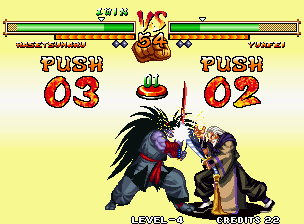;This is the news for this code.. eheheh now we have two ( 2 ) helper for the visual counter... to see how many times we have pushed the button ( unit.. 0,1,2,3,4 etc  and 10,20,30 etc )
[state 28000, Helper1]
type = Helper
triggerall = facing=1 ;IsHometeam
trigger1 = time = 0 && numhelper(28010)=0
helpertype = normal
name = "unità"
ID =28010
stateno = 28010
pos = 50,-20
postype = left
facing = 1
keyctrl = 0
ownpal = 1
sprpriority = 10

[state 28000, Helper2]
type = Helper
triggerall = facing=1 ;IsHometeam
trigger1 = time = 0 &&numhelper(28011)=0
helpertype = normal
name = "decimali"
ID =28011
stateno = 28011
pos = 40,-20
postype = left
facing = 1
keyctrl = 0
ownpal = 1
sprpriority = 10

[state 28000, Helper1a]
type = Helper
triggerall = facing= -1 ;!IsHometeam
trigger1 = time = 0 && numhelper(28010)=0
helpertype = normal ;player
name = "unità"
ID =28010
stateno = 28010
pos = -40,-20
postype =right
facing = 1
keyctrl = 0
ownpal = 1
sprpriority = 10

[state 28000, Helper2a]
type = Helper
triggerall = facing= -1 ;!IsHometeam
trigger1 = time = 0 && numhelper(28011)=0
helpertype = normal ;player
name = "decimali"
ID =28011
stateno = 28011
pos = -50,-20
postype =right
facing = 1
keyctrl = 0
ownpal = 1
sprpriority = 10

;Everytime the player push the button to this var is added 1point...
; IsHomeTeam is for the P1
; !IsHomeTeam is for P2 ( enemy )
[state 28000, 3a]
triggerall = IsHomeTeam
trigger1 = command = "a"
trigger2 = command = "b"
trigger3 = command = "c"
trigger4 = command = "x"
trigger5 = command = "y"
trigger6 = command = "z"
var(1) = 1

[state 28000, 3b]
triggerall = !IsHomeTeam
trigger1 = command = "a"
trigger2 = command = "b"
trigger3 = command = "c"
trigger4 = command = "x"
trigger5 = command = "y"
trigger6 = command = "z"
var(2)=1

;All this changestate are used to decide and define if we have:
;28003 = win
;28002 =lose
;120 =draw <- yes is a "block" state... You can change to "stand" or other custmon statedef

[state 28000, 4vince]
type = ChangeState
triggerall = IsHomeTeam
triggerall = time >= 200
trigger1 = var(1) > enemy,var(2)
value = 28003
ctrl = 0

[state 28000, 4perde]
type = ChangeState
triggerall = IsHomeTeam
triggerall = time >= 200
trigger1 = var(1) < enemy,var(2)
value = 28002
ctrl = 0

[state 28000, 4pareggia]
type = ChangeState
triggerall = IsHomeTeam
triggerall = time >= 200
trigger1 = var(1) = enemy,var(2)
value = 120
ctrl = 1

[state 28000, 4vince]
type = ChangeState
triggerall = !IsHomeTeam
triggerall = time >= 200
trigger1 = var(2) > enemy,var(1)
value = 28003
ctrl = 0

[state 28000, 4perde]
type = ChangeState
triggerall = !IsHomeTeam
triggerall = time >= 200
trigger1 = var(2) < enemy,var(1)
value = 28002
ctrl = 1

[state 28000, 4pareggia]
type = ChangeState
triggerall = !IsHomeTeam
triggerall = time >= 200
trigger1 = var(2) = enemy,var(1)
value = 120
ctrl = 1

;As said this is the transition state for the enemy hitted by our character.. from this custmonstatedef.. the enemy turn to the pushpush mode.
;P2 tmp
[Statedef 28001]
type    = S
movetype= H
physics = N
velset = 0,0

[State 28001 2]
type = SelfState
trigger1 = time = 1
value = 28000

;If we lose the challenge.. our character go to this statedef. for only 300gameticks.. during this time our character is dizzied ( if no anim 5300 found.. he play the stand animation ).
;LOSE state
[Statedef 28002]
type    = S
movetype= H
physics = N
velset = 0,0

[State 28002, ChangeAnim]
type = ChangeAnim
trigger1 = selfanimexist(5300)=1
value = 5300

[State 28002, ChangeAnim]
type = ChangeAnim
trigger1 = selfanimexist(5300)=0
value = 0

[State 28002, end]
type = SelfState
trigger1 = time >= 300
value = 5100

The 28003 statedef are left in to your hands... is the stadef for the winner of the pushpush mode... use your fantasy

;This is the code for one of the helper that show on the screen the number of times that you have pushed the buttons
;unità / unit
[StateDef 28010]
type = S
movetype= I
physics = S
anim = 28500
velset = 0,0
sprpriority = 10

[State 28010, VarSet]
type = VarSet
trigger1 = time = 0
v = 30
value = 0

;Every time the palyer push one of this button to the variable 30 is added 1 unit....
trigger1 = parent, command = "a"
trigger2 = parent, command = "b"
trigger3 = parent, command = "c"
trigger4 = parent, command = "x"
trigger5 = parent, command = "y"
trigger6 = parent, command = "z"
v = 30
value = 1

[State 28010, VarSet]
type = Varset
trigger1 =  var(30)>=10
v = 30
value = 0

[State 28010, ChangeAnim]
type = ChangeAnim
trigger1 = parent, command = "a"
trigger2 = parent, command = "b"
trigger3 = parent, command = "c"
trigger4 = parent, command = "x"
trigger5 = parent, command = "y"
trigger6 = parent, command = "z"
value = 28500+var(30)

[State 28010, end]
type = destroyself
trigger1 = parent,stateno !=28000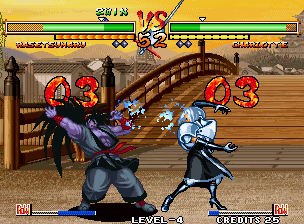Mathematic.. only this nothing of special
[StateDef 28011]
type = S
movetype= I
physics = S
velset = 0,0
anim = 28500
sprpriority = 10

[State 28011, VarSet]
type = VarSet
trigger1 = time = 0
v = 40
value = 0

trigger1 = parent, command = "a"
trigger2 = parent, command = "b"
trigger3 = parent, command = "c"
trigger4 = parent, command = "x"
trigger5 = parent, command = "y"
trigger6 = parent, command = "z"
v = 40
value = 1

[State 28011, ChangeAnim]
type = ChangeAnim
trigger1 = var(40) = 10
trigger2 = var(40) = 20
trigger3 = var(40) = 30
trigger4 = var(40) = 40
trigger5 = var(40) = 50
trigger6 = var(40) = 60
trigger7 = var(40) = 70
trigger8 = var(40) = 80
trigger9 = var(40) = 90
trigger10 = var(40) = 100
value = 28500+(var(40)/10)

[State 28011, end]
type = destroyself
trigger1 = parent,stateno !=28000

To let your character enter to the pushpush mode world, You need:
This file: esppc.cns <- in this file the are all the statedef for the pushpush code....
edit the statedef 280 ( is the starter ).. edit only some thing in the statedef 28000 ( I have quoted the part that you can modify )
And remember that you can redone as you wish the statedef 28003

AIR:

28000 pushpush animation for your character
[Begin Action 28000]
230,0, 0,0, 5
230,1, 0,0, 5
230,2, 0,0, 5
230,0, 0,0, 5
etc....

28001 big portrait
[Begin Action 28001]
9000,2, 0,0, 5

28002  figer that push the button
[Begin Action 28002]
6001,0, 0,0, 5
6001,1, 0,9, 5

from 28500 to 28509 are the counter's number
[begin action 28500]
6000,0, 0,0, 1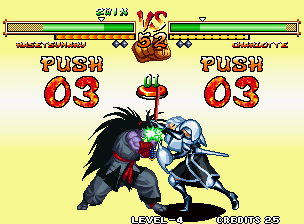;To help to identify your char as a "pushpush code char" in to the stadef -2 of your char put this:
[State -2, vs]
type = varset
trigger1 = 1
var(0) = 59

I have created the char "CAVIA" with the finger frames and all the numbers frame to let you start in a easy way

Probabily we will use this code in the Masters Project... but who know eheheh
by
Kain the supreme

You can see my code in actin on this:
Characters:
EvilSoul by kain the supreme http:www.kaints.com
Ken Washio by kain the supreme http:www.kaints.com
Jun Shiratori by kain the supreme http:www.kaints.com
Spider Girl by kain the supreme http:www.kaints.com

hentai character for adults only, 18+ only
Kyla Hentai by kain the supreme http://winmugenx.blogspot.com/

Full Game: